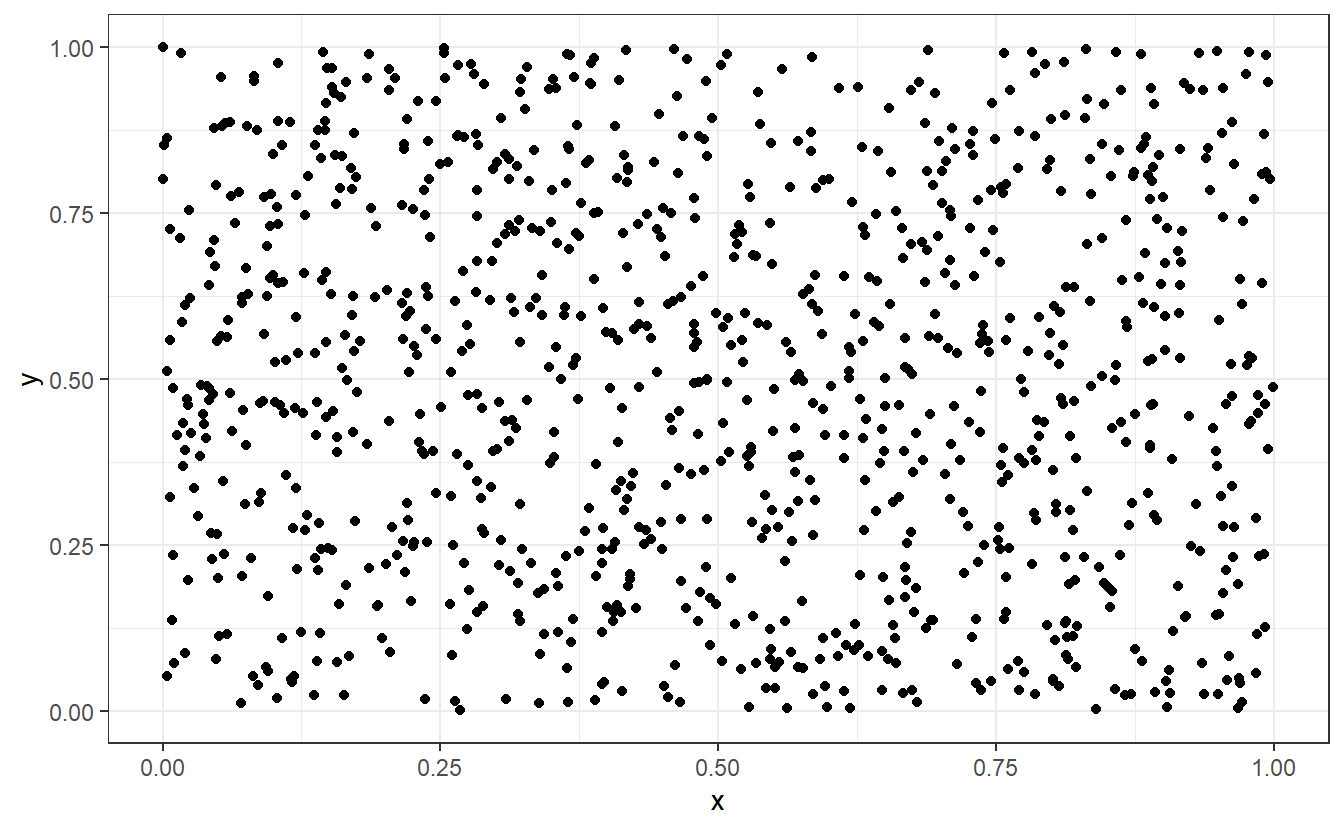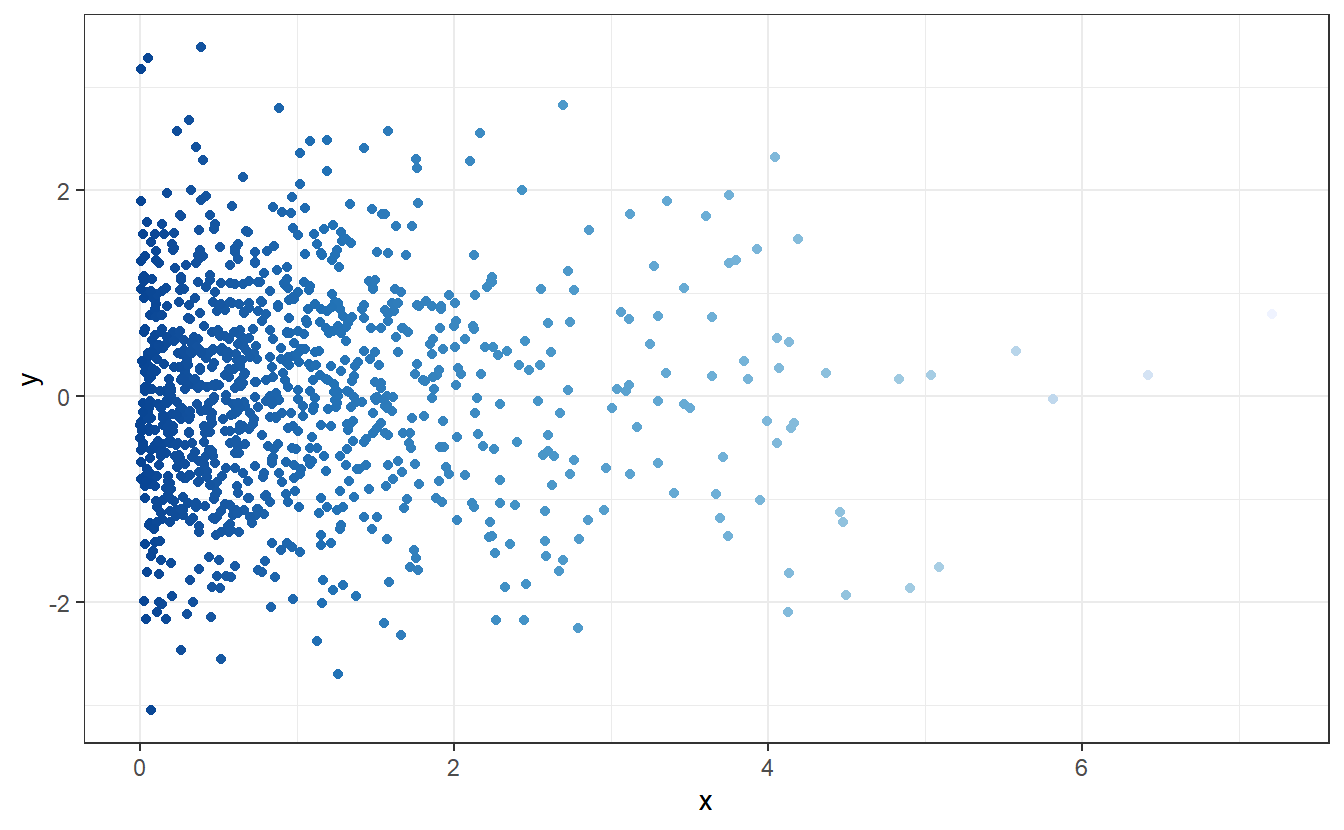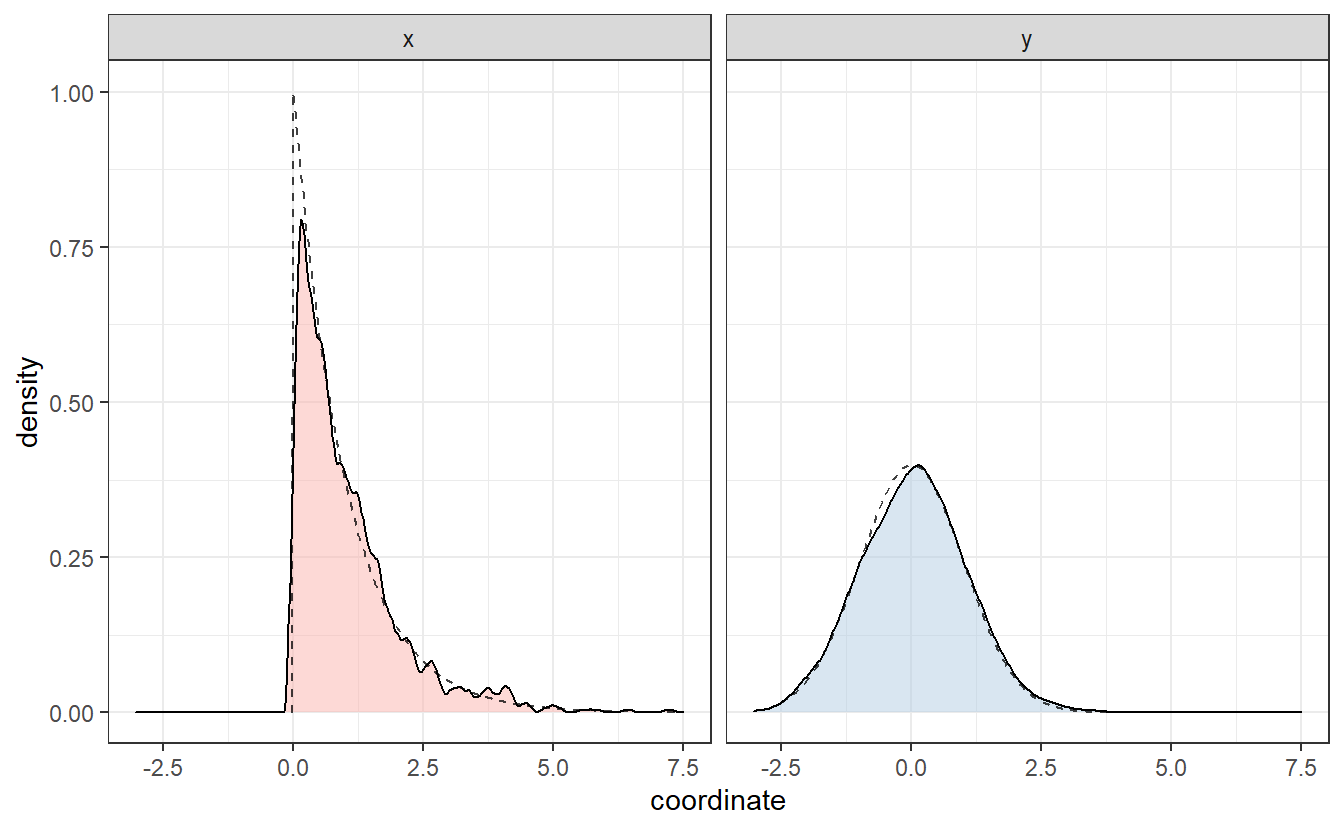# 4 Random Number Generators

In this chapter, we want to talk about randomness. Surely, if we want to extract meaning from data, we will have to differentiate between actual patterns and random fluctuations. Thus, a quick study of randomness is in order.

Of course, everything from philosophical to mathematical aspects of randomness cannot possibly be covered here. Therefore, let us focus on how to generate numbers that look convincingly random.

What do I mean by that? Imagine a scatter plot of a lot of randomly scattered points in the unit square, i.e. in $$[0, 1]^2$$. What you imagine may look like this.Wikipedia describes randomness as “the apparent lack of pattern or predictability in events”44 and intuitively, most people would probably say that this picture right here certainly fits that bill. However, you may (rightfully) suspect that this picture is not “truly” random in the sense that there is no definite way to recreate this picture. That is, of course, because there is a way to recreate this plot.

I simulated numbers between 0 and 1 to use as x- and y-coordinates of the points and the rest was only a matter of calling ggplot(). Naturally, this implies that there is a way to generate numbers that (hopefully) look the random part but are, in fact, anything but random because someone has to instruct R “what” to compute.

As we know from elementary probability classes, randomness can come in different shapes in the sense of different probability distributions. For instance, look at this new scatter plot:Here, the points look scattered all over the place45. This time, however, it looks like there seems to be at least some overall tendencies present. More specifically, it appears that there are way more points whose x- and y-coordinates are close to zero.

We can discern these patterns, if we look only at the x- and y-coordinates and their distribution. Same as we did in Chapter 2, we can plot an estimation of the corresponding densities via geom_density().This basically resembles what we have surmised from the scatter plot: In both coordinates, values close to zero are favored way more than others. Interestingly, these estimated densities look similar to the densities of an exponential resp. a Gaussian distribution (as seen by the dashed lines). These were in fact the distributions I was aiming to simulate in the second scatter plot.

Consequently, it is also possible to generate numbers that can pass of as realizations of an exponential or Gaussian distribution. In the course of this chapter, we will see that we can do that for many more distributions.

## 4.1 Simulating Uniformly Distributed Random Variables

The basis for many simulation techniques is the ability to simulate numbers that appear to be uniformly distributed on $$[0, 1]$$. As the introduction suggests, none of the simulated numbers are “truly random” which is why they are usually called pseudorandom numbers.

One of the most basic generator of pseudorandom numbers are so-called linear congruential generators (LCG). These generate numbers on a set $$E = \{ 0, \dots, m - 1\}$$ where $$m \in \mathbb{N}$$ is a given positive integer. The specific recursive generation procedure for a pseudorandom number $$x_n$$ is given by \begin{align*} x_{n+1} = (ax_n + c) \mod m, \end{align*} for fixed constants $$a, c \in \mathbb{N}$$. Dividing these numbers by $$m$$ will lead to numbers in $$[0, 1]$$.

Finally, the above recursion implies that we need some initial value $$x_0$$. This initial value is usually called seed and is often chosen as something “random”, e.g. decimal places of the generator’s starting time.

Note that the knowledge of the generator, i.e. the above recursion formula and the constants $$a, c$$ and $$m$$, make the pseudorandom numbers reproducible. Reproducibility can be of advantage if you are working with simulations so that other researches/coworkers can reproduce your findings. Also, it helps to compare for instance two procedures on the same pseudorandom numbers. Otherwise, you may never know if differences in the procedures are due to random fluctuations or a different performance of either procedure.

Let us implement a LCG with some initial parameters. To do so, we will have to learn about for-loops. Theses help us for sequential computations such as the above recursion. The general syntax of R’s for-loop looks like this

for (<loop index> in <loop range>) {
<instructions here>
}

In our case, this may look like this

# Coefficients of LCG
m <- 100
a <- 4
c <- 1
seed <- 5

# Create empty vector so that we can fill it with
# pseudorandom numbers
n <- 15
x <- numeric(n)
x <- seed

# Loop through recursion
for (i in 1:(n-1)) {
x[i + 1] <- (a * x[i] + c) %% m
}
x
#>    5 21 85 41 65 61 45 81 25  1  5 21 85 41 65

Dividing x by m would then lead to the desired numbers on $$[0, 1]$$. However, if you look at the sequence of numbers you may notice that there is evil afoot46. Obviously, there is some periodicity to the numbers which will not help us on our pursuit of randomness.

This periodicity may be eleviated by a “better” choice of the LCG parameters. One such choice leads to so called RANDU generator.

# RANDU coefficients
m <- 2^31
a <- 65539
c <- 0
seed <- 1 # any odd positive integer

# Create empty vector so that we can fill it with
# pseudorandom numbers
n <- 16
x <- numeric(n)
x <- seed

# Loop through recursion
for (i in 1:(n-1)) {
x[i + 1] <- (a * x[i] + c) %% m
}
x
#>            1      65539     393225    1769499    7077969   26542323
#>     95552217  334432395 1146624417 1722371299   14608041 1766175739
#>  1875647473 1800754131  366148473 1022489195
Now, this looks better but is it really? Let’s see what happens if we simulate more points and use 3 consecutive pseudorandom numbers as coordinates within the unit cube $$[0, 1]^3$$.